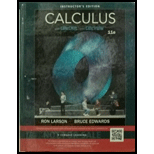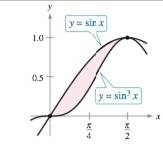Chapter 8.3, Problem 71E### Calculus (MindTap Course List)

11th Edition
Ron Larson + 1 other
ISBN: 9781337275347

#### Solutions

Chapter
Section### Calculus (MindTap Course List)

11th Edition
Ron Larson + 1 other
ISBN: 9781337275347
Textbook Problem

# Area In Exercises 71 and 72, find the area of the given region. y = sin x , y = sin 3 xTo determine

To calculate: The area of given region of the functions y=sinx , y=sin3x.

Explanation

Given: y=sinx , y=sin3x

Refer the image in the giving question.

Calculation:

Consider the area in the given region,

y=sinx , y=sin3x

Refer the image in the giving question.

From the graph, the limit of x = 0,π2. You can also find the limit of x by algebraic calculation.

Now let’s find the intersection point by equating y.

sinx=sin3xsinxsin3x=0sinx(1sin2x)=0

Simplify further,

sinx=0 or sin2x=1.

x=0 or x=π2

So that the limit of x are,

x=0 , π2

Hence the area of region is,

A=0π2(sinxsin3x)dx

Use the trigonometry identity,

sin3x=3sinx4sin3xsin3x=14(3sinxsin3x)

So,

A=0π2

### Still sussing out bartleby?

Check out a sample textbook solution.

See a sample solution

#### The Solution to Your Study Problems

Bartleby provides explanations to thousands of textbook problems written by our experts, many with advanced degrees!

Get Started

## Additional Math Solutions

#### Factor each expression in Problems 7-18 as a product of binomials. 14.

Mathematical Applications for the Management, Life, and Social Sciences

#### Draw the garph of y+2=-23x-1.

Elementary Geometry for College Students

#### In Exercises 110, find the graphical solution to each inequality. 3x+6y12

Finite Mathematics for the Managerial, Life, and Social Sciences